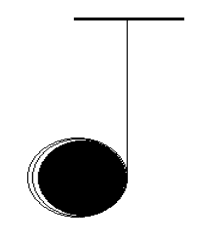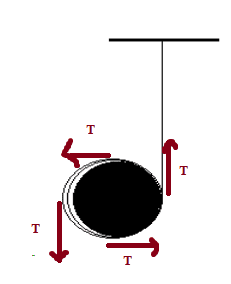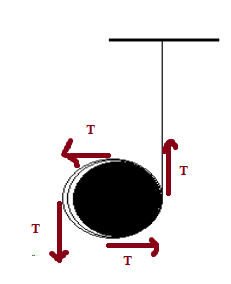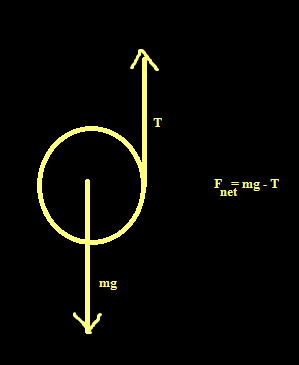# Tension in string

## Homework Statement[/B]
A string is wound around a uniform disk of radius ##10cm## and mass ##300g##. The
disk is released from rest when the string is vertical and its top end is tied to a
fixed bar. Determine the tension in the string. [ ##g = 10 ms^{-2} ##]

## The Attempt at a Solution

I think it is just ##300g \cdot 10 ms^{-2}## ;

haruspex
Homework Helper
Gold Member
2020 Award
I think it is just ##300g \cdot 10 ms^{-2}##
If so, what is the net vertical force on the disk? What acceleration would result?

If so, what is the net vertical force on the disk? What acceleration would result?
The accelaration of the disc is ##g## .But, the disc will rotate, I think. And there should be a torque. I shown in the picture, the tension of the string ## T## will create the torque.

haruspex
Homework Helper
Gold Member
2020 Award
The accelaration of the disc is gg .
The acceleration of the disk would be g if you took the string away. If there's any tension in the string the acceleration must be less.
Write the ##\Sigma F = ma## equation for the vertical direction.

The acceleration of the disk would be g if you took the string away. If there's any tension in the string the acceleration must be less.
Write the ##\Sigma F = ma## equation for the vertical direction.I shown in the picture, there is tension in the string, but the net force in the vertical direction is still ##mg## .

haruspex
Homework Helper
Gold Member
2020 Award
View attachment 78250
I shown in the picture, there is tension in the string, but the net force in the vertical direction is still ##mg## .
OK, I see where you are confused. There are different ways of treating how the portion of the string wrapped around the disk comes into it.
The least confusing is to assume it barely wraps around at all, i.e. as though it is attached to the disk at the point where they meet.
If you want to consider a longer wrap, it gets complicated because the least friction will soon result in its being effectively attached. If we say there's no friction at all then you have to follow it around to where it is ultimately attached, at some arbitrary point on the rim. T will apply tangentially there, but nowhere else. At all other parts of the string touching the disk, the force exerted by the string is radial. We can go down that path if you like, or you can trust me that it will all come out the same in the end... as though the string is attached to the disk at the point where they first meet.

T will apply tangentially there, but nowhere else.
But why?

haruspex
Homework Helper
Gold Member
2020 Award
But why?
If there's no friction how can the string apply a tangential force all along its length? It has no grip. Can you open a screw-top jar with greasy hands?

If there's no friction how can the string apply a tangential force all along its length? It has no grip. Can you open a screw-top jar with greasy hands?
I got your point. So, in this problem, the tension is simply 0.

Stephen Tashi
I got your point. So, in this problem, the tension is simply 0.

I suggest you follow the standard procedure and draw a correct free body diagram of the disk instead of the diagram you drew. Are you familiar with the concept of a free body diagram?

I suggest you follow the standard procedure and draw a correct free body diagram of the disk instead of the diagram you drew. Are you familiar with the concept of a free body diagram?
Yes.
I think it will be as I drawn below:And, ##F_{net} = ma## ; where, ##a## is the accelaration of the disc.
Will it be, ##a = g## ?

And I think there will be a torque acting on the disc, which is equal to ##Tr## ; where ##r## is the radius of the disc.
Again, torque = ##I \alpha## ; where, ##I## is moment of inertia and ##\alpha## is the angular accelaration;
So, ##Tr = \frac{1}{2} mr^2 \alpha##; because, moment of inertia of a disc with radius ##r##, is ##\frac {1}{2}mr^2##
##\alpha = \frac {2T}{mr}##
Again, ##a = r \alpha##
##a = r \frac {2T}{mr}##
##a = \frac {2T}{m} ##
And so,
##mg - T = ma = m \frac{2T}{m}##
##mg -T = 2T##
##T = \frac{1}{3} mg##

And I think there will be a torque acting on the disc, which is equal to ##Tr## ; where ##r## is the radius of the disc.
Again, torque = ##I \alpha## ; where, ##I## is moment of inertia and ##\alpha## is the angular accelaration;
So, ##Tr = \frac{1}{2} mr^2 \alpha##; because, moment of inertia of a disc with radius ##r##, is ##\frac {1}{2}mr^2##
##\alpha = \frac {2T}{mr}##
Again, ##a = r \alpha##
##a = r \frac {2T}{mr}##
##a = \frac {2T}{m} ##
And so,
##mg - T = ma = m \frac{2T}{m}##
##mg -T = 2T##
##T = \frac{1}{3} mg##

That looks correct.:)